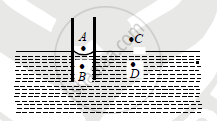HSC Science (General) 12th Board ExamMaharashtra State Board
Share

Books Shortlist

# Explain the Rise of Liquid in the Capillary on the Basis of Pressure Difference. - HSC Science (General) 12th Board Exam - Physics

ConceptCapillarity and Capillary Action

#### Question

Explain the rise of liquid in the capillary on the basis of pressure difference.

#### Solution

Explanation of capillary action: Suppose that a capillary tube is dipped in a liquid like water which wets the capillary. Let us consider the situation before the movement of water inside the capillary. The shape of the surface in the capillary is concave. Let us consider four points A, B, C, D such that (i) A be just above the curved surface inside the capillary. (ii) B is just be low inside the capillary. (iii) C is just above the plane surface outside the capillary. (iv) D is just below the plane surface outside the capillary PA, PB, PC, PD be the pressures at these points.As the pressure on the concave side of the liquid surface is greater than convex sides PA>P....(1)

As the pressure is smae on the both side of a plane surface PC=PD ......(2)

Now PA=PC = atmospheric pressure ......(3)

From relation (1), (2) and (3) PD >PB

But B and D are same horizonal level in liquid, where pressure at D is greater than pressure at B. Therefore liquid cannot remain in equilibrium and it flows in to the capillary and rises above B till the pressure at B and D becomes equal. This is the reason why where is rise of liquid inside the capillary tube.

Is there an error in this question or solution?

#### APPEARS IN

2013-2014 (March) (with solutions)
Question 1.1 | 2.00 marks

#### Video TutorialsVIEW ALL 

Solution Explain the Rise of Liquid in the Capillary on the Basis of Pressure Difference. Concept: Capillarity and Capillary Action.
S